# III Electron Structure A Energy Levels Energy levels

• Slides: 12III Electron Structure A Energy Levels • Energy levels are distances from the nucleus where electrons can be found – there are 7 energy levels » 1 st is the lowest energy and closest to nucleus » Each level holds a maximum number of electrons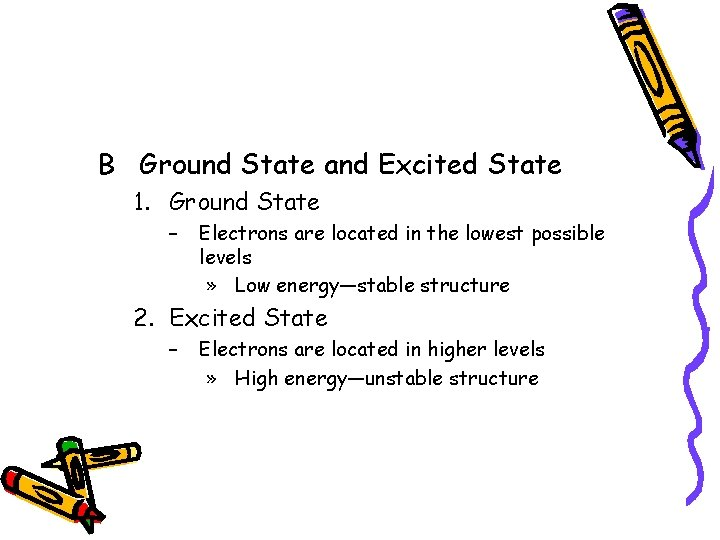B Ground State and Excited State 1. Ground State – Electrons are located in the lowest possible levels » Low energy—stable structure 2. Excited State – Electrons are located in higher levels » High energy—unstable structure3. Emission Spectra – When an atom in the ground state absorbs energy, electrons get excited » These excited electrons, “jump” to a higher energy level [excited state] » When electrons return to ground state, they release energy in the form of light » Light pattern is unique for each element » Color is related to each electron transition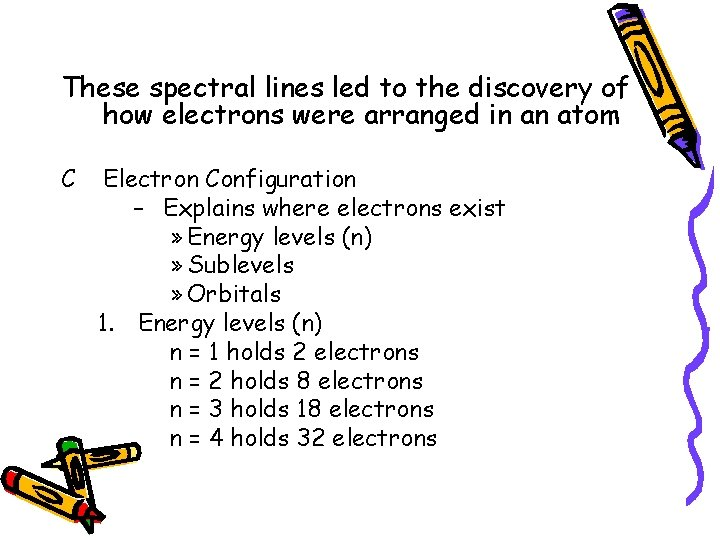These spectral lines led to the discovery of how electrons were arranged in an atom C Electron Configuration – Explains where electrons exist » Energy levels (n) » Sublevels » Orbitals 1. Energy levels (n) n = 1 holds 2 electrons n = 2 holds 8 electrons n = 3 holds 18 electrons n = 4 holds 32 electrons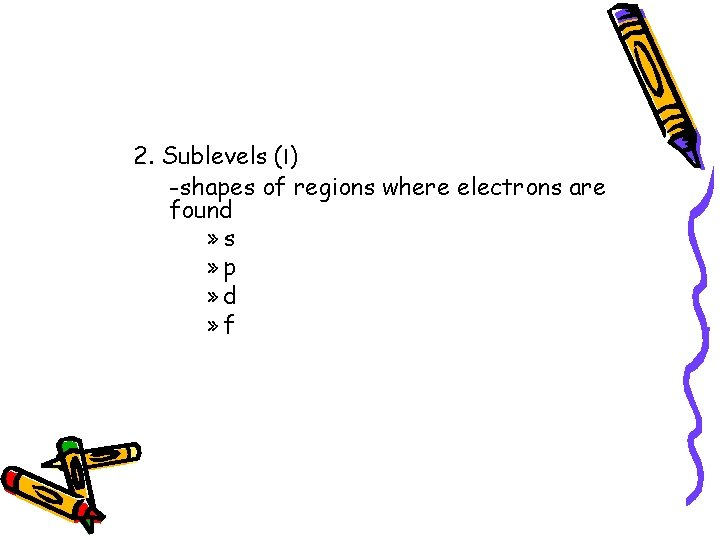2. Sublevels (l) -shapes of regions where electrons are found » s » p » d » f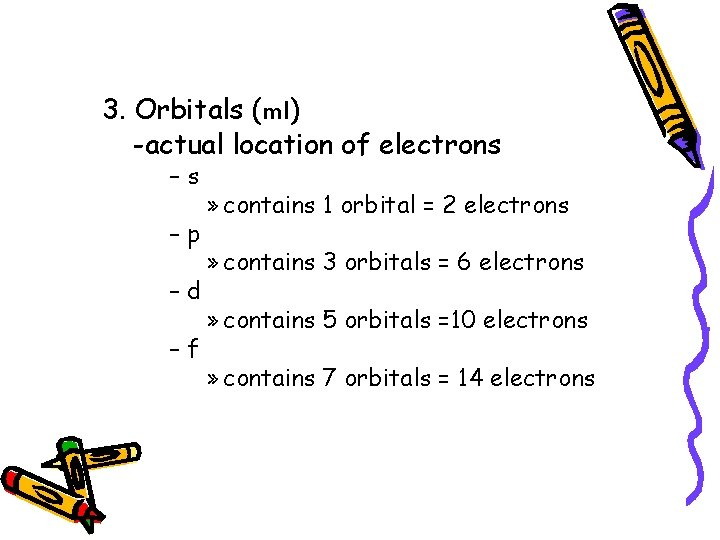3. Orbitals (ml) -actual location of electrons –s –p –d –f » contains 1 orbital = 2 electrons » contains 3 orbitals = 6 electrons » contains 5 orbitals =10 electrons » contains 7 orbitals = 14 electrons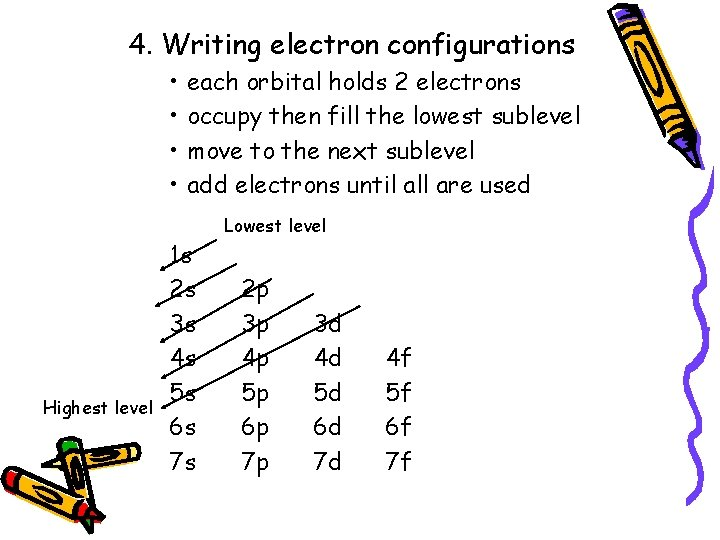4. Writing electron configurations • • Highest level each orbital holds 2 electrons occupy then fill the lowest sublevel move to the next sublevel add electrons until all are used 1 s 2 s 3 s 4 s 5 s 6 s 7 s Lowest level 2 p 3 p 4 p 5 p 6 p 7 p 3 d 4 d 5 d 6 d 7 d 4 f 5 f 6 f 7 f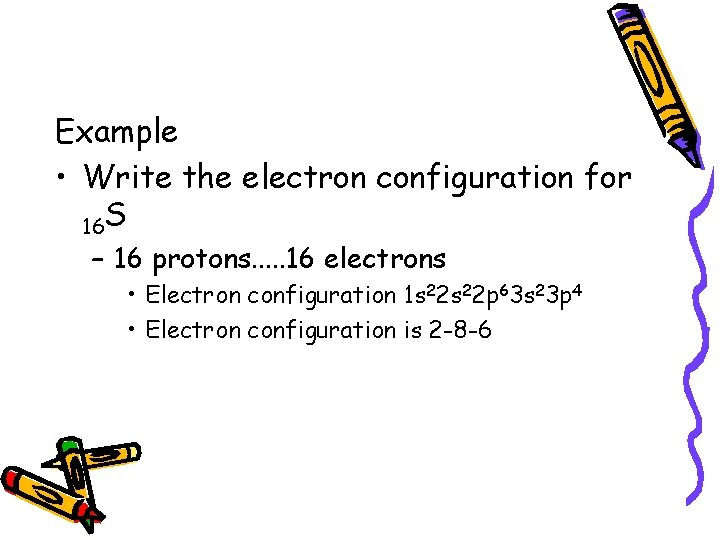Example • Write the electron configuration for 16 S – 16 protons. . . 16 electrons • Electron configuration 1 s 22 p 63 s 23 p 4 • Electron configuration is 2 -8 -6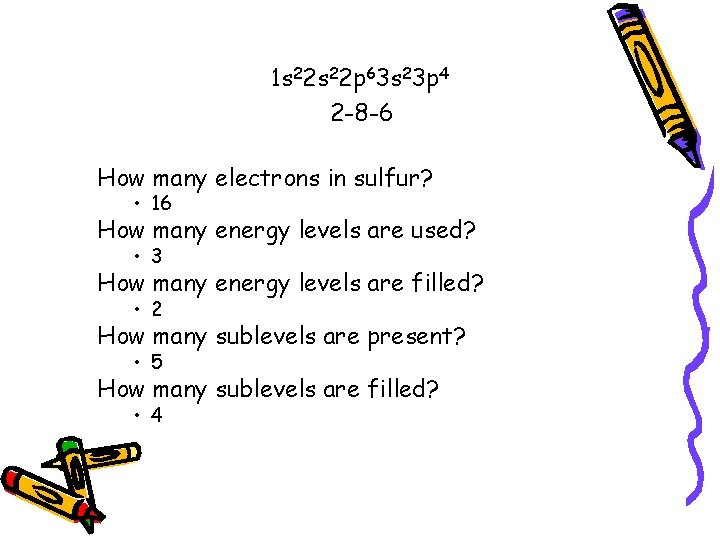1 s 22 p 63 s 23 p 4 2 -8 -6 How many electrons in sulfur? • 16 How many energy levels are used? • 3 How many energy levels are filled? • 2 How many sublevels are present? • 5 How many sublevels are filled? • 4Write the electron structure for: 1. 2. 3. 4. 5. 6. 7. 8. 9. 10. 11 Na 6 C 33 As 17 Cl 3 Li 13 Al 10 Ne 35 Br 18 Ar 8 O• • • Na = 1 s 22 p 63 s 1 C = 1 s 22 p 2 As = 1 s 22 p 63 s 23 p 64 s 23 d 104 p 3 Cl = 1 s 22 p 63 s 23 p 5 Li = 1 s 22 s 1 Al = 1 s 22 p 63 s 23 p 1 Ne = 1 s 22 p 6 Br = 1 s 22 p 63 s 23 p 64 s 23 d 104 p 5 Ar = 1 s 22 p 63 s 23 p 6 O = 1 s 22 p 4